# During 2022, Ayya Comp. reported net sales of \$2000000, net income of 5800000, and depreciation expense...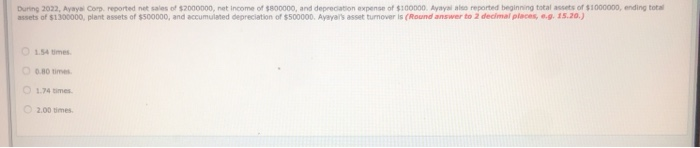During 2022, Ayya Comp. reported net sales of \$2000000, net income of 5800000, and depreciation expense of \$100000. Ayay reported beginning total assets of \$1000000, ending total assets of \$1300000 plant assets of \$500000, and accumulated depreciation of \$500000. Ayaya a t turnover is (Round answer to 2 decimal places, .g. 15.20.) 1.54 times 0.80 times 1.74 times 2.00 times

Working

 Asset Turnover A Sales \$        2,000,000.00 B Beginning Balance of Total Assets \$        1,000,000.00 C Ending Balance of Total Assets (see working #1) \$        1,300,000.00 D = (B+C)/2 Average Total Assets \$        1,150,000.00 E= A/D Asset Turnover 1.74

#### Earn Coin

Coins can be redeemed for fabulous gifts.

Similar Homework Help Questions
• ### During 2022, Blue Spruce Corp. reported net sales of \$2000000, net income of \$870000, and depreciation...

During 2022, Blue Spruce Corp. reported net sales of \$2000000, net income of \$870000, and depreciation expense of \$140000. Blue Spruce also reported beginning total assets of \$1080000, ending total assets of \$1460000, plant assets of \$510000, and accumulated depreciation of \$700000. Blue Spruce’s asset turnover is (Round answer to 2 decimal places, e.g. 15.20.) a ) 0.80 times. b 1.37 times. c 1.57 times. d 1.85 times.

• ### During 2017, Flint Corporation reported net sales of \$2000000, net income of \$860000, and depreciation expense...

During 2017, Flint Corporation reported net sales of \$2000000, net income of \$860000, and depreciation expense of \$150000. Flint also reported beginning total assets of \$1010000, ending total assets of \$1340000, plant assets of \$590000, and accumulated depreciation of \$750000. Flint’s asset turnover (rounded) is a. 1.70 times. b. 1.98 times c. 0.78 times. d. 1.49 times.

• ### please answer 13,14,15 13 During 2022, Phelps Corporation reported not sales of \$2.500.000 not income of...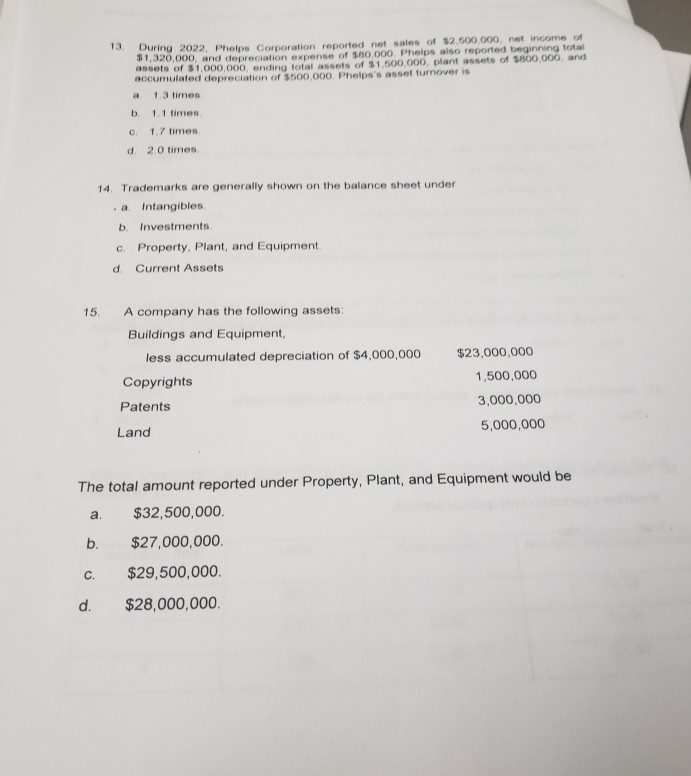please answer 13,14,15 13 During 2022, Phelps Corporation reported not sales of \$2.500.000 not income of 31,320,000, and depreciation pense of \$80.000 Phelps also reported beginning total assets of \$1,000,000 onding total assets of \$1.500.000 plant assets of SB00.000. and accumulated depreciation of \$500.000 Phelps's assol turnover is a 1.3 times b. 11 times C 1.7 times d 2.0 times 14. Trademarks are generally shown on the balance sheet under . a Intangibles b. Investments c Property. Plant, and Equipment...

• ### Please show me how to calculate this problem: During 2007, Sitter Corporation reported net sales of \$2,000,000, net income of \$1,200,000, and depreciation expense of \$100,000

Please show me how to calculate this problem: During 2007, Sitter Corporation reported net sales of \$2,000,000, net income of \$1,200,000, and depreciation expense of \$100,000. Sitter also beginning total asset of \$1,000,000, ending total asset of \$1,500,000, plant asset of \$800,000, and accumulated depreciation of \$500,000. Sitter’s asset turnover ratio is:

• ### Suppose during 2022 that Federal Express reported the following information (in millions): net sales of \$34,750...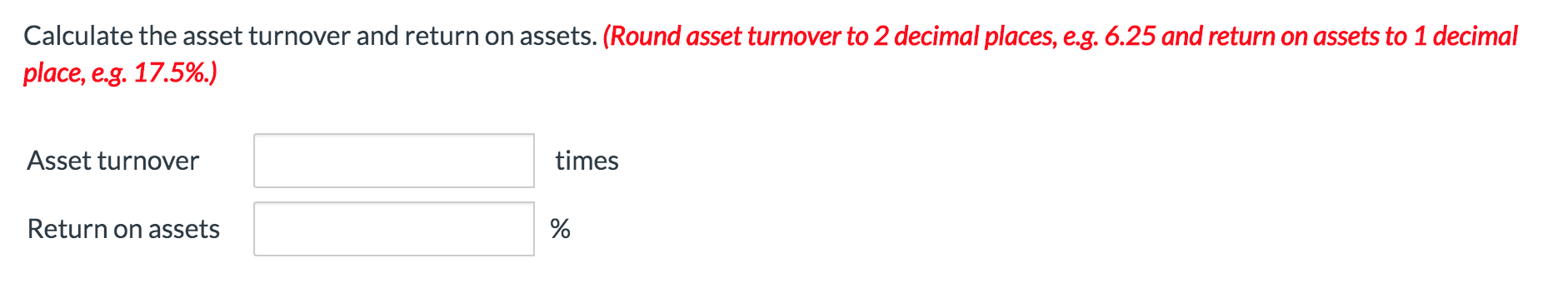Suppose during 2022 that Federal Express reported the following information (in millions): net sales of \$34,750 and net income of \$95. Its balance sheet also showed total assets at the beginning of the year of \$24,650 and total assets at the end of the year of \$23,300. Calculate the asset turnover and return on assets. (Round asset turnover to 2 decimal places, e.g. 6.25 and return on assets to 1 decimal place, e.g. 17.5%.) Asset turnover times Return on assets

• ### – Your answer is partially correct. Suppose during 2022 that Federal Express reported the following information...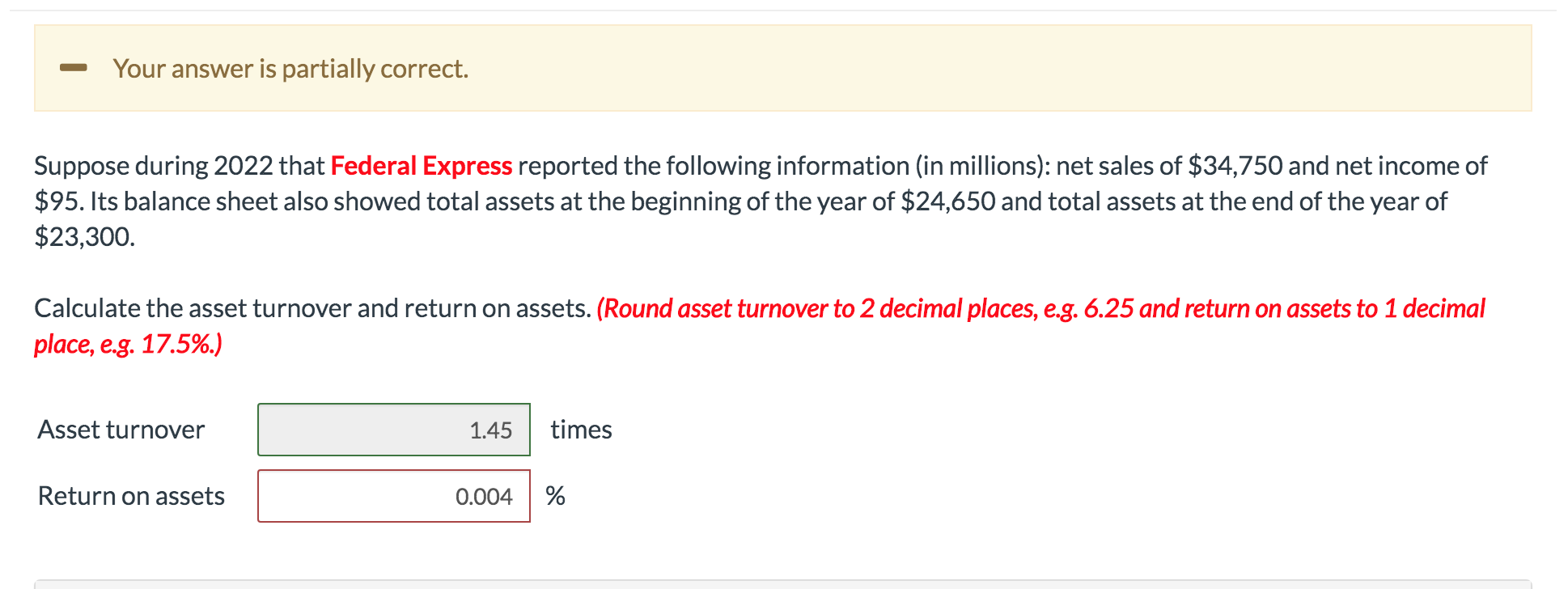– Your answer is partially correct. Suppose during 2022 that Federal Express reported the following information (in millions): net sales of \$34,750 and net income of \$95. Its balance sheet also showed total assets at the beginning of the year of \$24,650 and total assets at the end of the year of \$23,300. Calculate the asset turnover and return on assets. (Round asset turnover to 2 decimal places, e.g. 6.25 and return on assets to 1 decimal place, e.g. 17.5%.)...

• ### Brad's BBQ reported sales of \$780,000 and net income of \$32,500. Brad’s also reported ending total...

Brad's BBQ reported sales of \$780,000 and net income of \$32,500. Brad’s also reported ending total assets of \$499,000 and beginning total assets of \$392,000. Required: Calculate the return on assets, the profit margin, and the asset turnover ratio for Brad's BBQ.

• ### Brad's BBQ reported sales of \$810,000 and net income of \$35,500. Brad's also reported ending total...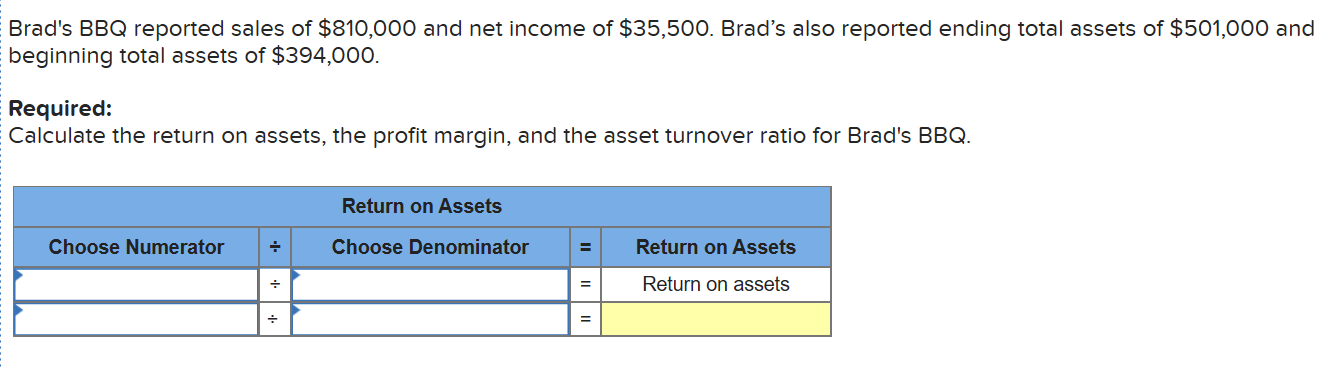Brad's BBQ reported sales of \$810,000 and net income of \$35,500. Brad's also reported ending total assets of \$501,000 and beginning total assets of \$394,000. Required: Calculate the return on assets, the profit margin, and the asset turnover ratio for Brad's BBQ. Return on Assets Choose Numerator - Choose Denominator Return on Assets Return on assets .

• ### Analyze Fixed Assets At December 31, 2013, Clark Corporation reported beginning net fixed assets of \$94,150,...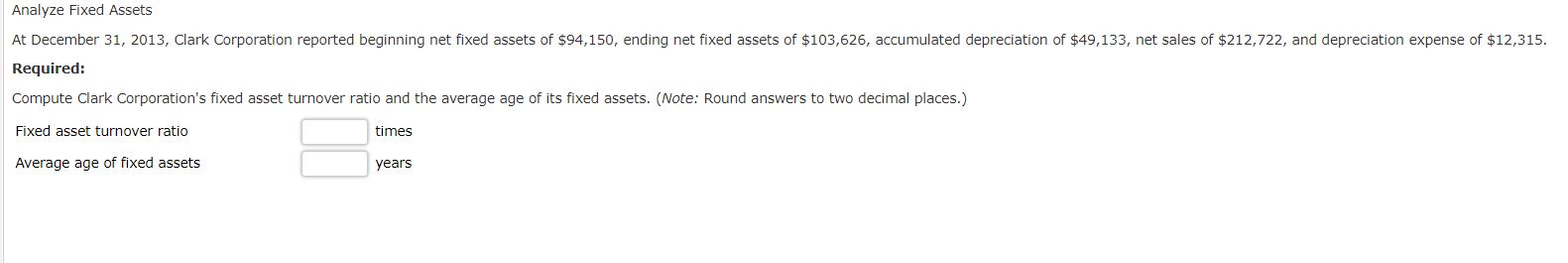Analyze Fixed Assets At December 31, 2013, Clark Corporation reported beginning net fixed assets of \$94,150, ending net fixed assets of \$103,626, accumulated depreciation of \$49,133, net sales of \$212,722, and depreciation expense of \$12,315. Required: Compute Clark Corporation's fixed asset turnover ratio and the average age of its fixed assets. (Note: Round answers to two decimal places.) Fixed asset turnover ratio times Average age of fixed assets years

• ### The 2021 income statement for Anderson TV and Appliance reported net sales of \$360,000 and net...

The 2021 income statement for Anderson TV and Appliance reported net sales of \$360,000 and net income of \$85,000. Average total assets for 2021 was \$800,000. Shareholders’ equity at the beginning of the year was \$500,000 and \$40,000 was paid to shareholders as dividends. There were no other shareholders’ equity transactions that occurred during the year. Show the DuPont framework's calculation of the three components of the 2021 return on equity for Anderson TV and Appliance. Profit Margin (%) Choose...